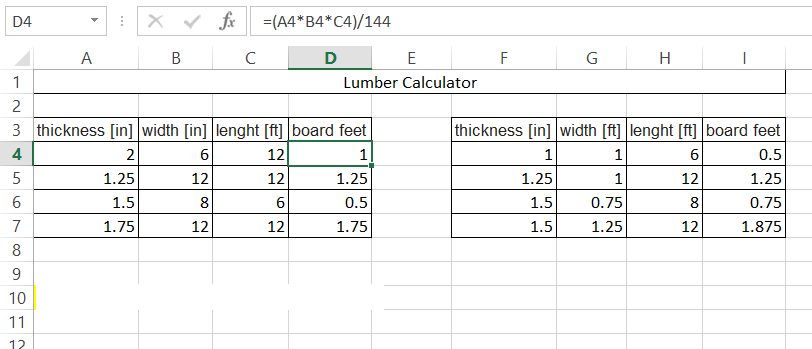## Board Footage Calculator in Excel

In this Excel lesson you will teach yourself how to prepare Board Footage Calculator. Board footage is a volume of hardwoodlumber (to buy).1 board foot equals one square foot of wood which is one inch thick. Here is the relation:1 board foot = 144 cubic inches = 1/12 cubic foot To prepare such lumber calculations you need to prepare data first. You will need:

• board length [feet]
• width [ft or inches]
• thickness [in]
`In case you prefer metric units you may need cm to inches converter.`

To calculate wood board footage you just need to multiply length * width * thickness.

Board foot formula = length * width * thickness

To adjust units you need to use this formulas:

board foot = length(ft) * width(in) * thickness(in) / 144board foot = length(ft) * width(ft) * thickness(in) / 12Now you know how to calculate board feet.

## Template

```Further reading: